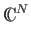Next: Dirac Notation: Up: State Vectors and Dirac Previous: State Vectors and Dirac   Contents

#### State Vectors:

We can describe the state of any quantum system by a state vector in a Hilbert space. A Hilbert space is a complex linear vector space. In the Hilbert space for a state vector describing an N-state quantum system there will be N perpendicular axes, which correspond to the measurable states of the system. These are called basis states or eigenstates. In general, the total state of a quantum system can be any complex linear combination of the basis states. The Hilbert space for a single qubit has two perpendicular axes, one corresponding to the 0 state, and the other corresponding to the 1 state.Next: Dirac Notation: Up: State Vectors and Dirac Previous: State Vectors and Dirac   Contents
Matthew Hayward Lower Query Bounds in the Quantum Oracle Model GitHub Repository